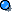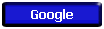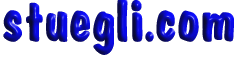##### California State Standards - Physics Grades 9-12

##### Conservation of Energy and Momentum

2. The laws of conservation of energy and momentum provide a way to predict and describe the movement of objects. As a basis for understanding this concept:

 a. Students know how to calculate kinetic energy using the formula E=(1/2)mv2. b. Students know how to calculate changes in gravitational potential energy near Earth by using the formula (change in potential energy) = mgh (h is the change in the elevation). c. Students know how to solve problems involving conservation of energy in simple systems, such as falling objects. d. Students know how to calculate momentum as product mv. e. Students know momentum is a separately conserved quantity different from energy. f. Students know an unbalanced force on an object produces a change in its momentum. g. Students know how to solve problems involving elastic and inelastic collisions in one dimension by using the principles of conservation of momentum and energy. h. * Students know how to solve problems involving conservation of energy in simple systems with various sources of potential energy, such as capacitors and springs.Copyright © 2005 -  S. B. EglI